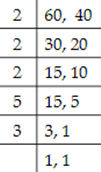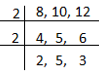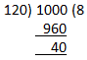# CBSE Class 6 Mathematics Chapter 3 Important Questions## myCBSEguide

Trusted by 70 Lakh Students

CBSE Class 6 Mathematics Chapter 3 Important Questions. myCBSEguide has just released Chapter Wise Question Answers for class 6 Maths. There chapter wise Practice Questions with complete solutions are available for download in myCBSEguide website and mobile app. These Extra Questions with solution are prepared by our team of expert teachers who are teaching grade in CBSE schools for years. There are around 4-5 set of solved Mathematics Extra questions from each and every chapter. The students will not miss any concept in these Chapter wise question that are specially designed to tackle Exam. We have taken care of every single concept given in CBSE Class 6 Mathematics syllabus and questions are framed as per the latest marking scheme and blue print issued by CBSE for Class 6.

CBSE Class 6 Maths Extra Questions

## Extra Questions of Class 8 Maths Playing with Numbers

Ch – 3 Playing with Numbers

1. Which of them is not a prime number?
1. 23
2. 4
3. 11
4. 13
2. _____ is the factor of 68.
1. 17
2. 6
3. 3
4. 5
3. The sum of two odd and one even numbers is
1. Even of odd
2. Odd
3. even number
4. Prime
4. Which of the following is divisible by 3?
1. 15287
2. 15267
3. 152638
4. 15286
5. The number of multiples of a given number is _______.
1. None of these
2. infinite
3. 2
4. finite
6. Match the following:

Column IColumn II
a. 15p. Factor of 50
b. 12q. Factor of 30
c. 25r. Factor of 65
d. 13s. Factor of 48
7. Fill in the blanks:

1. 1 is neither _____ nor _____.
2. The smallest prime number is _____.
3. The smallest composite number is _____.
4. the smallest even number is _____.
8. State whether the following statements are True or False.

1. A number with 4 or more digits is divisible by 8, if the number formed by the last three digits is divisible by 8.
2. If the sum of the digits of a number is divisible by 3, then the number itself is divisible by 9.
3. All numbers which are divisible by 4 may not be divisible by 8.
4. The Highest Common Factor of two or more numbers is greater than their Lowest Common Multiple.
9. Find LCM of 60 and 40.
10. Write all the factors of the following number : 27
11. List all the multiples of 7 that lie between 125 and 142.
12. Find the H.C.F of the following numbers. 70, 105, 175
13. Find the H.C.F of the following numbers. 27, 63
14. Find all the multiples of 9 upto 100.
15. Find the least 4-digit number which is exactly divisible by 8, 10, and 12.

Ch – 3 Playing with Numbers

1. 4
Explanation: 4 is not a prime number as it has more than 2 factors.
1. 17
Explanation: 68 = 2 x 2 x 17. Therefore 17 is the factor of 68.
1. even number
Explanation: The sum of two odd numbers is always a even number.
Sum of two even numbers is even number. So when two odd numbers and one even number added is even number.
For ex. 1 + 3 = 4 (even number) and 6 + 4 = 10 (even number)
1. 15267
Explanation: 15267 is correct response.
If u Add the digits of the number I.e 1+5+2+6+7=21, the sum is the multiple of 3. Therefore, the number is divisible by 3
1. infinite
Explanation: The multiple of any number is infinite as a number can multiplied till infinite
1. – q
2. – s
3. – p
4. – r
1. 1 is neither prime number nor composite number.
2. The smallest prime number is 2.
3. The smallest composite number is 4.
4. The smallest even number is 2.
1. True
2. False
3. True
4. False
1.LCM {tex} = {2^3} \times 3 \times 5 = 120{/tex}
2. 27 = 1 × 27
27 = 3 × 9
Thus, all the factors of 27 are 1, 3, 9 and 27.
3. By checking all the numbers from 125 to 142 which are divisible by 7 , we get 126, 133, 140.
So, the multiples of 7 that lie between 125 to 142 are 126, 133 and 140.
4. Factors of 70 are 1, 2, 5, 7, 10, 14, 35 and 70.
Factors of 105 are 1, 3, 5, 7, 15, 21, 35 and 105.
Factors of 175 are 1, 5, 7, 25, 35 and 175.
∴ Common factors of 70, 105 and 175 are 1,5,7 and 35.
Highest of these common factors is 35.
∴ H.C.F of 70, 105 and 175 is 35.
5. Factors of 27 are 1, 3, 9 and 27.
Factors of 63 are 1, 3, 7, 9, 21 and 63.
∴ Common factors of 27 and 63 are 1, 3 and 9.
Highest of these common factors is 9.
∴ H.C.F. of 27 and 63 is 9.
6. The multiples of 9 are
9 × 1 = 9 9 × 2=18 9 × 3= 27 9 × 4= 36 9 × 5=45 9 × 6=54 9 × 7 = 63 9 × 8 = 72 9 × 9= 81 9 × 10= 90 9 × 11=99 9 × 12= 108
Since 108 is greater than 100 therefore all the multiples of 9 upto 100 are 9, 18, 27, 36, 45, 54, 63, 72, 81, 90 and 99.
7. The smallest 4-digit number is 1000.
The least number which is exactly divisible by 8, 10, and 12 will be their L.C.M.
L.C.M. of 8, 10, and 12:L.C.M. of 8, 10, 12 = 2 × 2 × 2 × 5 × 3 = 120.
By dividing smallest 4-digit number 1000 by 120,We find that 1000 is not exactly divisible by 120.
If we subtract 40 from 1000, we get 1000 – 40 = 960, which will be divisible by 120. But 960 is a 3-digit number. The next number to 960 which is divisible by 120 is 960 + 120 = 1080.
Therefore, the required 4-digit number divisible by 8, 10, and 12 is 1080.

## Chapter Wise Extra Questions for Class 6 Mathematics## Test Generator

Create Tests with your Name & Logo## Job Openings at myCBSEguide

We are hiring Subject Matter Experts and Video Content Creators for our Dwarka, New Delhi office.